# Purpose:

The purpose of this wiki is to show that there can be differences between the values of HRA exemption calculated as per the older logic of Annual HRA (used in INHRA) and the new logic of Monthly HRA (used in INMHR), especially when there are master data changes in the middle of the financial year.

# Overview:

The explanation given here will cover Actual Projection Method (constant SLPRJ in the view V_T511K is set to ‘0’), however, the difference in calculation can also be observed if you’re using Nominal Projection.

## Master Data for first period:

Here, we’ll see the master data values for the first period of the financial year of the employee.  First up, we’ll see the Rent value that’s saved in IT0581.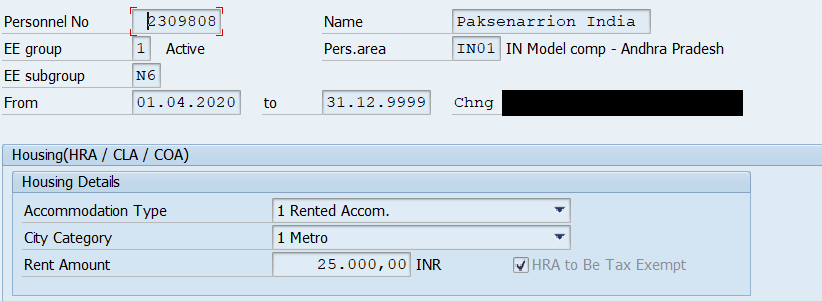Next, the value of HRA and Basic Pay that we’ve saved in IT0008 is as follows,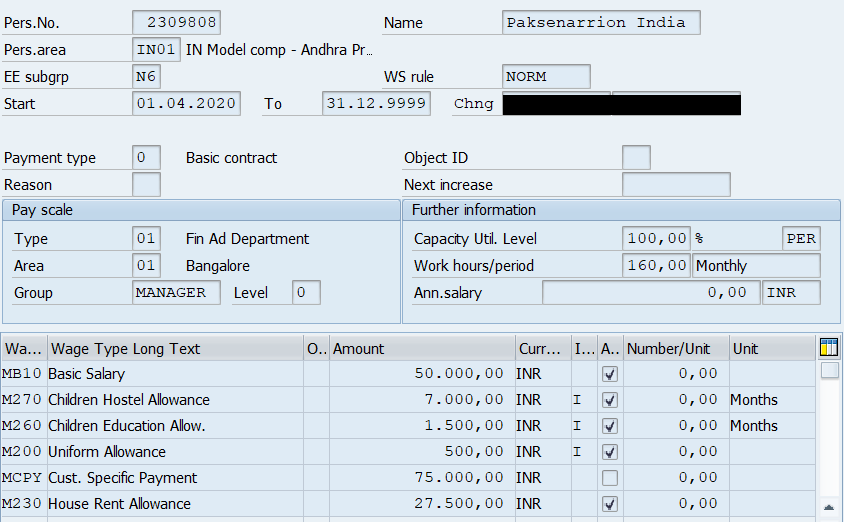## Processing in INHRA for first period:

Now, we can see the following in INHRA’s Processing node inside the payroll log for payroll run of April 2020: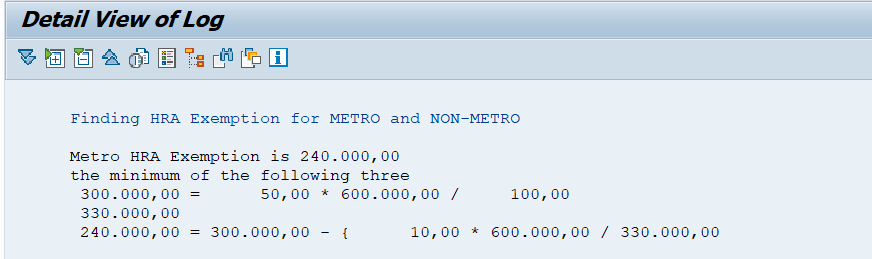Here, the three factors for HRA exemption are calculated as follows:

1.  50% of HRA basis = 0.50 * (50000 + (50000 * 11)) = 300,000
2. HRA Paid = 27500 + (27500 * 11) = 330,000
3. Rent Paid – 10% of HRA basis = (25000 * 12) – 0.10 * (50000 + (50000 * 11) = 240,000

The minimum of the three factors above would be INR 240,000.  Thus, the value of HRA exemption calculated in April 2020 by INHRA would be INR 240,000.

## Processing in INMHR for first period:

Now, if we check the processing in INMHR’s Processing node for this same employee inside the payroll log for April 2020, we’ll see,Here, the three factors for HRA exemption are calculated as follows:

1. 50% of HRA basis (monthly component) = 0.50 * 50000 = 25,000
2. HRA Paid (monthly component) = 27,500
3. Rent Paid – 10% of HRA basis (monthly components) = 25000 – (0.10 * 50000) = 20,000

The minimum of the three factors above would be INR 20,000.  However, this is only the monthly value for April 2020.  The total HRA exemption for the entire year will be calculated by using this same value and projecting it for the rest of the Financial Year.  The calculation would be as follows,

Annual HRA = 20000 + (20000 * 11) = 240,000

Thus, the value of HRA exemption calculated in April 2020 by INMHR would be INR 240,000.

Thus, in this case the values calculated in INHRA and INMHR are will be the same even though they use distinctly separate logics to arrive at their values.  However, master data changes are very common in the middle of the Financial Year, therefore, we’ll now see what happens when we input some master data changes.

## Master Data for second period:

Here, we’ll see the master data values for the second period of the financial year.  The scenario is that the values have been changed and no longer resemble the ones we entered earlier for the first period.  Therefore, the Rent value in IT0581 has been changed as follows: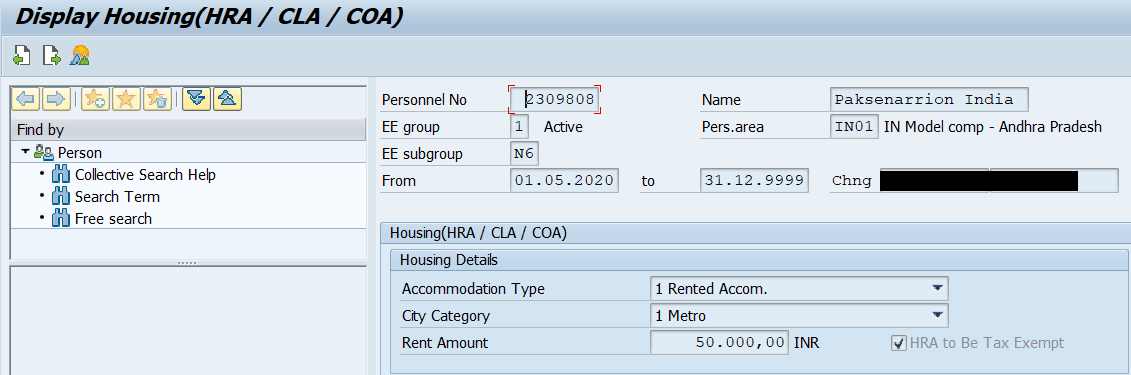Similarly, the master data in IT0008 has been changed as follows: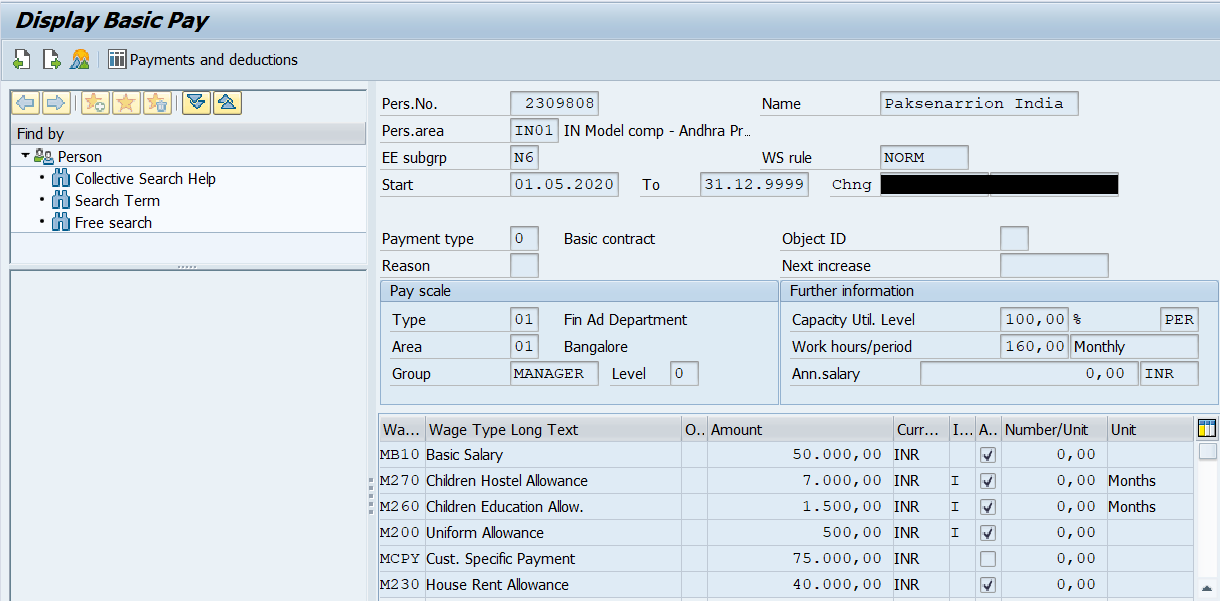## Processing in INHRA for second period:

Now, we can see the following in INHRA’s Processing node inside the payroll log for payroll run of May 2020: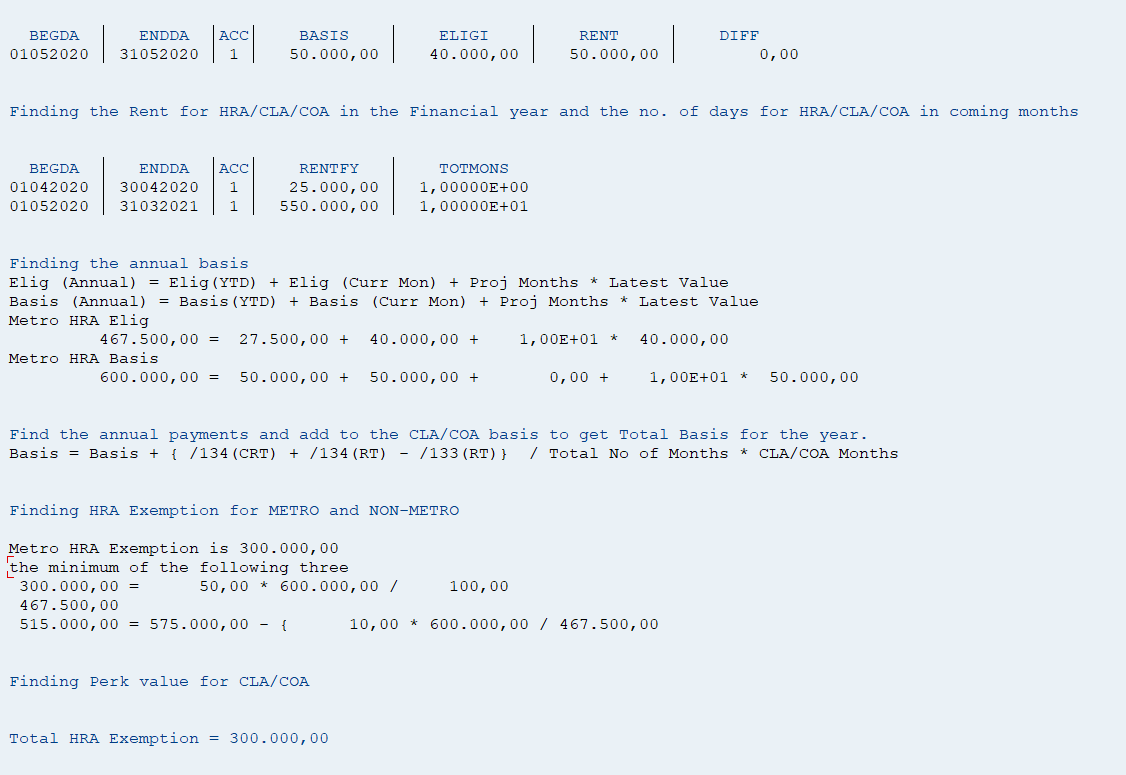Here, the three factors for HRA exemption are calculated as follows:

1.  50% of HRA basis = 0.50 * (YTD + current month’s value + projected value for rest of the year) = 0.50 * (50000 + 50000 + (50000 * 10)) = 300,000
2. HRA Paid = YTD + current month’s value + projected value for rest of the year = 27500 + 40000 + (40000 * 10) = 467,500
3. Rent Paid – 10% of HRA basis = (YTD Rent Paid + current month’s rent + projected value for the rest of the year) – 0.10 * (YTD HRA basis + current month’s basis + projected value for the rest of the year) = (25000 + (50000 * 11)) – 0.10 * (50000 + 50000 + (50000 * 10) = 515,000

The minimum of the three factors above would be INR 300,000.  Thus, the value of HRA exemption calculated in May 2020 by INHRA would be INR 300,000.

## Processing in INMHR for second period:

Now, if we check the processing in INMHR’s Processing node for this same employee inside the payroll log for May 2020, we’ll see,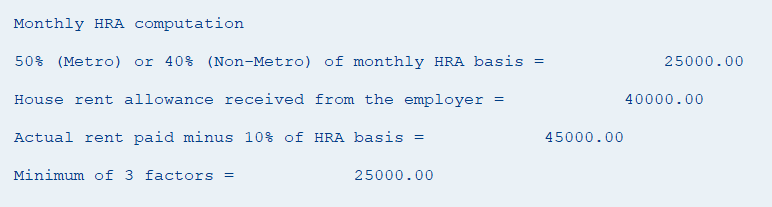Here, the three factors for HRA exemption are calculated as follows:

1. 50% of HRA basis (monthly component) = 0.50 * 50000 = 25,000
2. HRA Paid (monthly component) = 40,000
3. Rent Paid – 10% of HRA basis (monthly components) = 50000 – (0.10 * 50000) = 45,000

The minimum of the three factors above would be INR 25,000.  However, this is only the monthly value for May 2020.  The total HRA exemption for the entire year will be calculated by using the YTD value of HRA monthly exemption which will be added to the current month’s value and the current month’s value will be projected for the rest of the Financial Year.  The calculation would be as follows,

Annual HRA = 20000 + 25000  + (25000 * 10) = 295,000

Thus, the value of HRA exemption calculated in April 2020 by INMHR would be INR 295,00.  This is also shown in the screenshot below: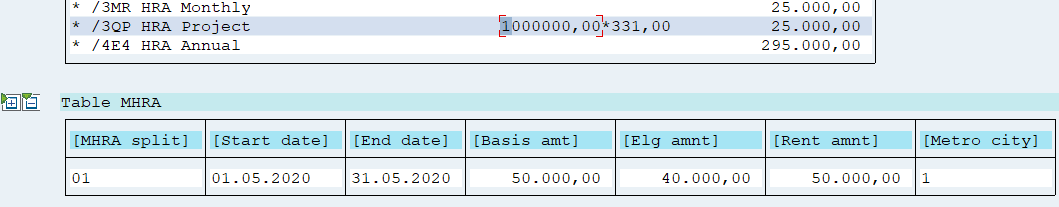/3MR is the current month’s HRA exemption component.

/3QP is the value of HRA exemption that is used to project the HRA for the rest of the year.

Thus, here we can see that the values of HRA calculation in INHRA and INMHR are now slightly different.  This is an expected behavior as the logic of these two functions is NOT the same.

IMPORTANT NOTE – INHRA will be sunset soon and will not be supported.  It is recommended that customers switch to using INMHR as soon as possible and explain the change in the logic to their business users to avoid any confusion down the line.